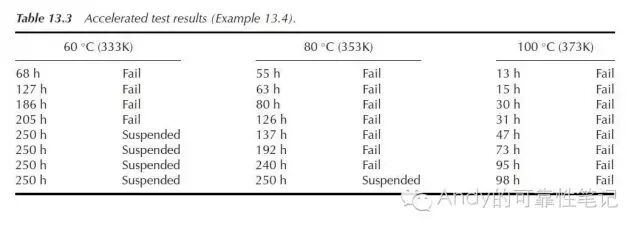# 可靠性加速寿命测试_Arrhenius 模型&Ea(激活能）取Ea:激活能；

k:Boltzmann常数=8.617×10-5ev/k；

Tu:产品正常使用下的开尔文温度，例如常温25度，则Tu=25+273=298K

Ts: 产品加速寿命测试时的环境应力温度；Tu=55+273=328K，Ts=125+273=398;

Ea=0.7（TI公司默认取通用值0.7，下面会提到）T=10478565360，α=1-60%=0.4（可接受的风险数）,2k+2=2(失效数为0）；代入上图单侧置信区间定时截尾方案的公式，得出MTBF=11435855069，约为1.1436*10的10次方，TI采用四舍五入直接取整为1*10的10次方。取24个电子器件，分为3组，分别在60度，80度，100度的条件下进行测试，测试最多进行到250h截止，若中间有失效也不返回修复。通过上图，可得出产品平均寿命（Mean Life)如下:

60度时平均寿命=(68+127+186+205+250+250+250+250)/4=396.5 h;-------①

80度时平均寿命=(55+63+80+126+137+192+240+250）/7=163.2857 h;---②

100度时产品平均寿命=（13+15+30+31+47+73+95+98）/8=50.25 h.-------③

Life=Aexp{Ea/(kT)},这里A为常量，T为开尔文温度，k为Boltzmann常数=8.617×10-5ev/k，对两边取自然对数得到如下公式：

Ln(Life)=LnA+Ea/(kT),-----④

Ln(396.5)=LnA+(Ea/k)*(1/333);---------⑤

Ln(163.2857)=LnA+(Ea/k)*(1/353);----⑥

Ln(50.25)=LnA+(Ea/k)*(1/373);---------⑦留言与评论（共有 0 条评论）

 验证码：